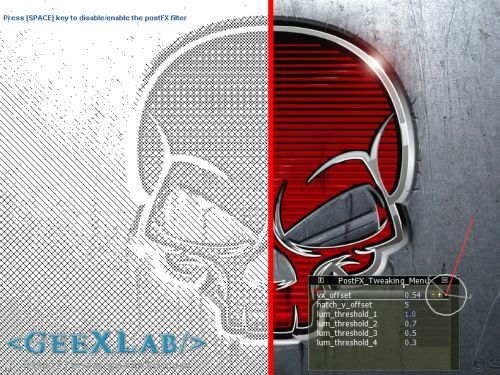# (Shader Library) Crosshatching GLSL FilterAs usual, while I was googling for some OpenCL stuff, I found something else: a simple cross-hatching pixel shader. Then I quickly coded a GeeXLab demo to play with.

What is the cross-hatching? From wikipedia:

Hatching (hachure in French) is an artistic technique used to create tonal or shading effects by drawing (or painting or scribing) closely spaced parallel lines. (It is also used in monochromatic heraldic representations to indicate what the tincture of a “full-colour” emblazon would be.) When lines are placed at an angle to one another, it is called cross-hatching.

You need GeeXlab 0.2.10 or better to run this demo. Start GeeXLab and load or drag and drop DEMO.xml in GeeXLab. That’s all.

I only tested this demo on my ASUS HD 6950 + Catalyst 11.2. Let me know if you encounter problems with other cards (especially NVIDIA boards).

Here is the complete cross-hatching GLSL shader for GeeXLab (or another app that support GLSL shaders):

```[Vertex_Shader]
void main(void)
{
gl_Position = ftransform();
gl_TexCoord = gl_MultiTexCoord0;
}

uniform sampler2D sceneTex; // 0
uniform float vx_offset;
uniform float rt_w; // GeeXLab built-in
uniform float rt_h; // GeeXLab built-in
uniform float hatch_y_offset; // 5.0
uniform float lum_threshold_1; // 1.0
uniform float lum_threshold_2; // 0.7
uniform float lum_threshold_3; // 0.5
uniform float lum_threshold_4; // 0.3
void main()
{
vec2 uv = gl_TexCoord.xy;

vec3 tc = vec3(1.0, 0.0, 0.0);
if (uv.x < (vx_offset-0.005))
{
float lum = length(texture2D(sceneTex, uv).rgb);
tc = vec3(1.0, 1.0, 1.0);

if (lum < lum_threshold_1)
{
if (mod(gl_FragCoord.x + gl_FragCoord.y, 10.0) == 0.0)
tc = vec3(0.0, 0.0, 0.0);
}

if (lum < lum_threshold_2)
{
if (mod(gl_FragCoord.x - gl_FragCoord.y, 10.0) == 0.0)
tc = vec3(0.0, 0.0, 0.0);
}

if (lum < lum_threshold_3)
{
if (mod(gl_FragCoord.x + gl_FragCoord.y - hatch_y_offset, 10.0) == 0.0)
tc = vec3(0.0, 0.0, 0.0);
}

if (lum < lum_threshold_4)
{
if (mod(gl_FragCoord.x - gl_FragCoord.y - hatch_y_offset, 10.0) == 0.0)
tc = vec3(0.0, 0.0, 0.0);
}
}
else if (uv.x>=(vx_offset+0.005))
{
tc = texture2D(sceneTex, uv).rgb;
}

gl_FragColor = vec4(tc, 1.0);
}
```

Background image: Red Skull (metallic).

References:

## 4 thoughts on “(Shader Library) Crosshatching GLSL Filter”

1.Michael

Cool, thanks for sharing

2.mg

Cool, thanks! Quick comment: You probably want to use a better estimate for luminance than length(rgb) – see e.g. http://en.wikipedia.org/wiki/YUV

3.mc

L = r*0.2126 + g*0.7152 + b*0.0722 gives you a reasonable approximation